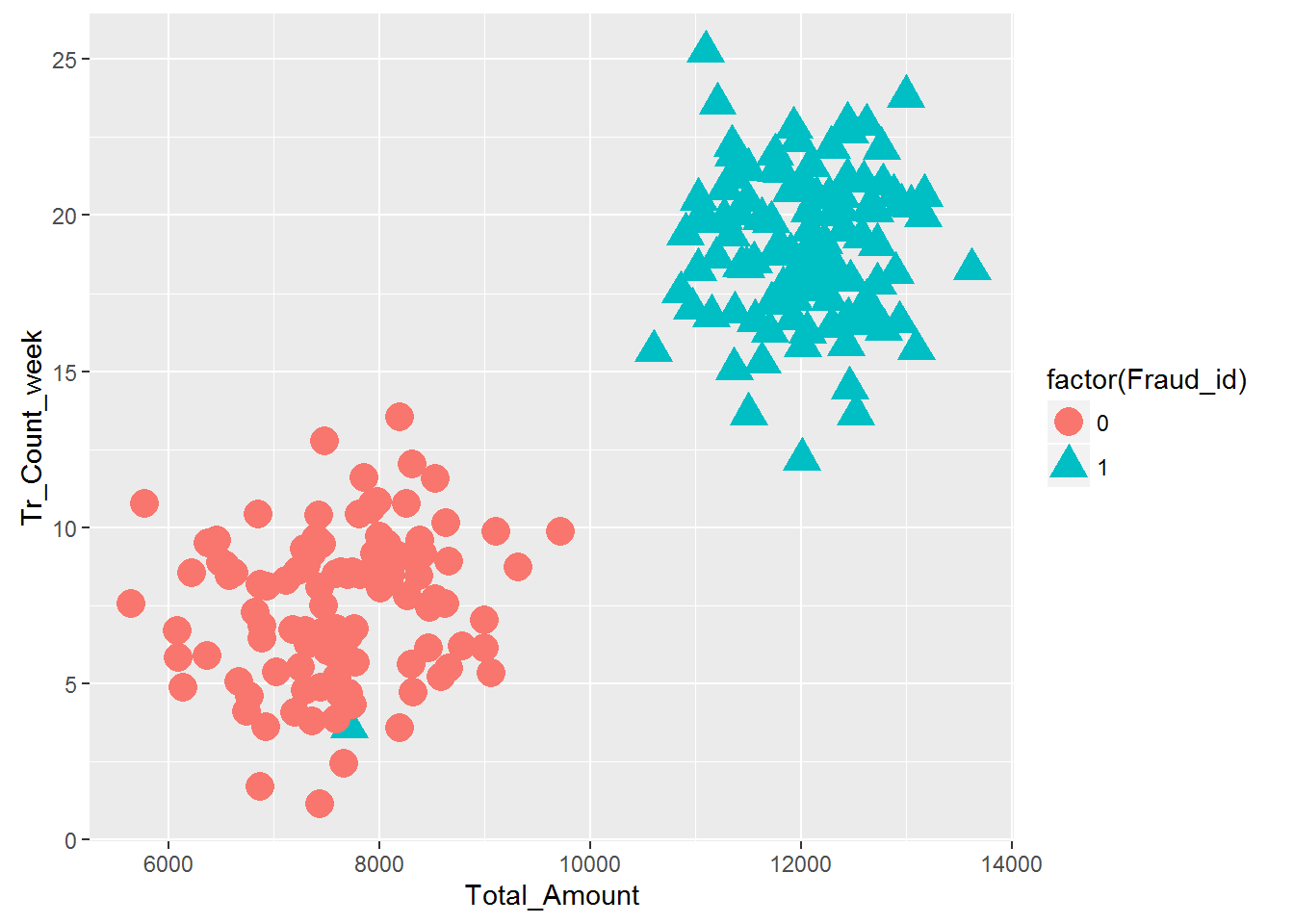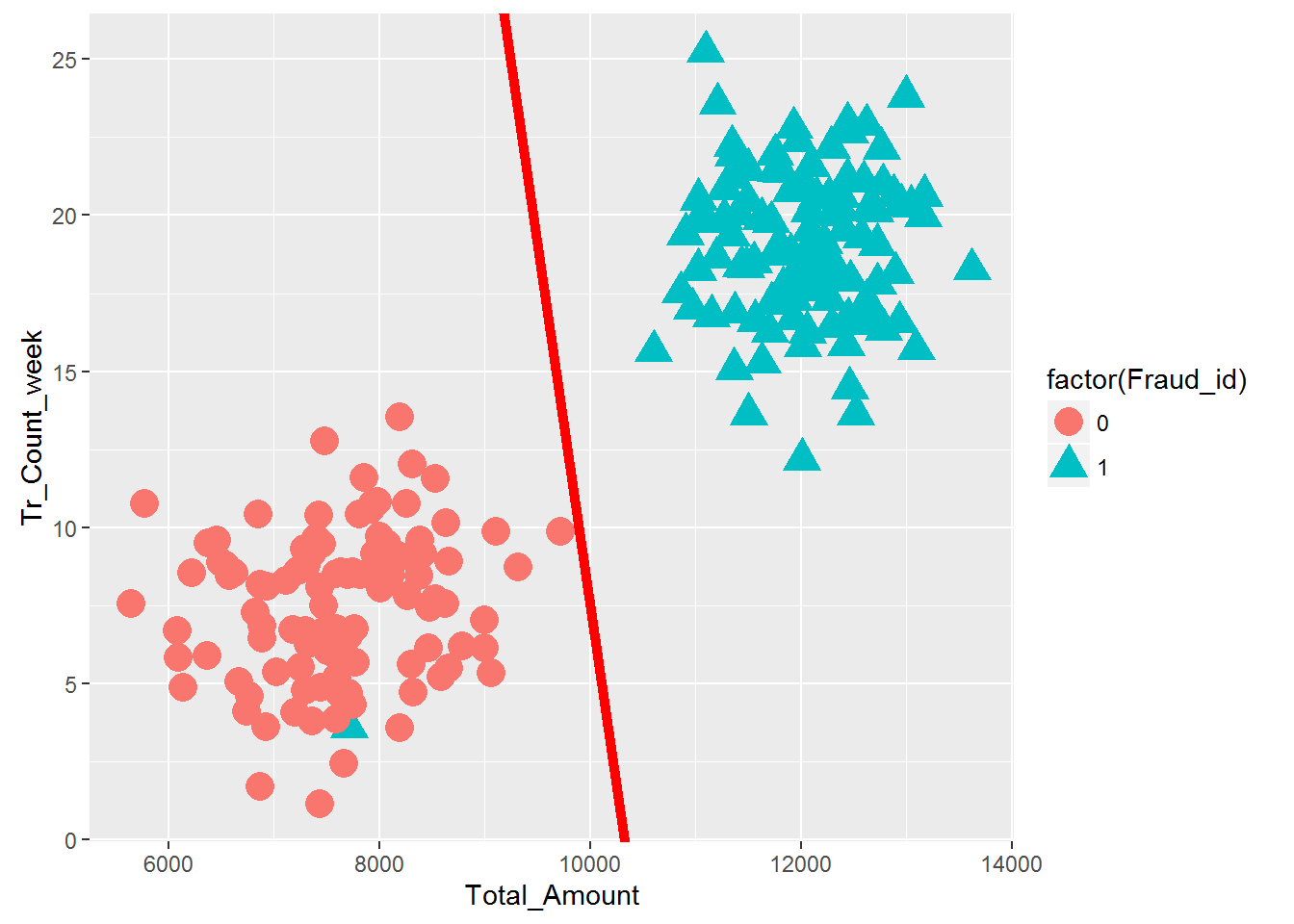• No products in the cart.

# 203.6.2 Practice : Simple Classifier

##### Let's put classifiers into practice

In previous section, we studied about Introduction to SVM

In this practice session we will cover all the things we discussed about simple classifiers in the last post.

### LAB: Simple Classifiers

• Dataset: Fraud Transaction/Transactions_sample.csv
• Draw a classification graph that shows all the classes
• Build a logistic regression classifier
• Draw the classifier on the data plot

### Solution

Transactions_sample <- read.csv("C:\\Amrita\\Datavedi\\Fraud Transaction\\Transactions_sample.csv")
head(Transactions_sample)
##      id Total_Amount Tr_Count_week Fraud_id
## 1 16078      7294.60          4.79        0
## 2 41365      7659.53          2.45        0
## 3 11666      8259.29         10.77        0
## 4 11824     11630.25         15.29        1
## 5 36414     12286.63         22.18        1
## 6    90     12783.34         16.34        1
names(Transactions_sample)
##  "id"            "Total_Amount"  "Tr_Count_week" "Fraud_id"
library(ggplot2)
ggplot(Transactions_sample)+geom_point(aes(x=Total_Amount,y=Tr_Count_week,color=factor(Fraud_id),shape=factor(Fraud_id)),size=5)#####Logit model

logit_model<-glm(Fraud_id~Total_Amount+Tr_Count_week,data=Transactions_sample,family=binomial())
logit_model
##
## Call:  glm(formula = Fraud_id ~ Total_Amount + Tr_Count_week, family = binomial(),
##     data = Transactions_sample)
##
## Coefficients:
##   (Intercept)   Total_Amount  Tr_Count_week
##    -26.148132       0.002534       0.108896
##
## Degrees of Freedom: 209 Total (i.e. Null);  207 Residual
## Null Deviance:       291.1
## Residual Deviance: 16.85     AIC: 22.85
###The classifier slope & intercept
coef(logit_model)
##   (Intercept)  Total_Amount Tr_Count_week
## -26.148131643   0.002533707   0.108895819
coef(logit_model)
## (Intercept)
##   -26.14813
coef(logit_model)
## Total_Amount
##  0.002533707
coef(logit_model)
## Tr_Count_week
##     0.1088958
logit_slope <- coef(logit_model)/(-coef(logit_model))
logit_intercept<- coef(logit_model)/(-coef(logit_model))

###The classifier diagram

base<-ggplot(Transactions_sample)+geom_point(aes(x=Total_Amount,y=Tr_Count_week,color=factor(Fraud_id),shape=factor(Fraud_id)),size=5)
base+geom_abline(intercept = logit_intercept , slope = logit_slope, color = "red", size = 2)The next post is about SVM Algorithm.

21st June 2017

### 0 responses on "203.6.2 Practice : Simple Classifier"

Statinfer Software Solutions LLP

Software Technology Parks of India,
NH16, Krishna Nagar, Benz Circle,